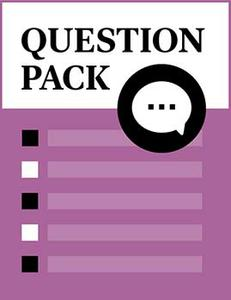# Computer Aided Design I

Source: Saylor

Student Price: FREE

This question pack asks students to apply their knowledge of computer aided design from the beginning.

Question 1

A working drawing consists of which of the following?

A

Detailed drawings

B

Assembly drawing

C

Bill of materials

D

All of the above

Question 2

Allowance is negative in which of the following fits?

A

Clearance fit

B

Transition fit

C

Interference fit

D

Both B and C

Question 3

Allowance is positive in which of the following fits?

A

Clearance fit

B

Transition fit

C

Interference fit

D

None of the above

Question 4

Auxiliary view is used to show what type of shape?

A

Oblique shape

B

True shape

C

Both A and B

D

None of the above

Question 5

Section lines should be oriented at ______________ for different parts.

A

Same angles

B

Different angles

C

90 degrees

D

30 degrees

Question 6

Clearance is always negative in which of the following fits?

A

Clearance fit

B

Transition fit

C

Interference fit

D

Both B and C

Question 7

Drawing information normally contains which of the following?

A

Part number

B

Part name

C

Revision number

D

All of the above

Question 8

A pictorial view shows ______________ dimensions in a single view.

A

One

B

Two

C

Three

D

Four

Question 9

An orthographic view shows ______________ dimensions in a single view.

A

One

B

Two

C

Three

D

Four

Question 10

Cutting plane lines in section view are shown using ______________ lines.

A

Thick continuous lines

B

Dashed lines

C

Center lines

D

Phantom Lines

Question 11

Cylindrical parts are generally shown using ______________ principle views.

A

One

B

Two

C

Three

D

Four

Question 12

Depth is drawn to full size in a ______________ projection.

A

Cavalier

B

Cabinet

C

Perspective

D

Trimetric

Question 13

Depth is drawn to half size in a ______________ projection.

A

Cavalier

B

Cabinet

C

Perspective

D

Trimetric

Question 14

Hidden features in CAD are shown using ______________ lines.

A

Thick continuous

B

Dashed

C

Center

D

Solid

Question 15

Primary auxiliary view is perpendicular to ______________ principle plane.

A

One

B

Two

C

Three

D

Four

Question 16

Sketching is usually the ______________ step in CAD drawing.

A

First

B

Second

C

Third

D

Last

Question 17

The difference between the maximum and minimum size limits on a part is ______________.

A

Allowance

B

Deviation

C

Clearance

D

Tolerance

Question 18

Visible features in CAD are shown using ______________ lines.

A

Thick continuous

B

Dashed

C

Center

D

Dotted

Question 19

First angle projections are associated with what types of units?

A

English units

B

International System of Units (SI)

C

Both A and B

D

None of the above

Question 20

Front view is drawn in true shape in which of the following projections?

A

Perspective projections

B

Isometric projections

C

Oblique projections

D

None of the above

Question 21

Hidden lines are often omitted in which of the following?

A

Detailed drawings

B

Assembly drawing

C

Bill of materials

D

All of the above

Question 22

How many principle views are there?

A

Three

B

Five

C

Six

D

None of the above

CC BY 3.0 - Saylor